### Homework exercise

To be solved at home before the exercise session.

1. Visit the website https://datavizproject.com/ and pick one data visualization/plot that interests you. Find out how it is drawn and what aspects of the data the different components represent. Be prepared to explain how your visualization of choice works in the class.

2. Type the command data() in R to show all data sets currently available in your installed packages. Go through the data sets and pick one that interests you. Check the help file of the data set using the command ?packagename for more detailed information. Be prepared to describe your answers to the following questions in the class:
• What is the purpose of the data? What kind of phenomenon does it describe?
• What kind of study is behind the data (observational, controlled, simulation, survey or something else)?
• How is the data represented in R (univariate, multivariate, time series…)?
• What kind of plots would you use to best summarize the data?
• What kind of numerical statistics would you use to best summarize the data?

### Class exercise

To be solved at the exercise session.

Note: all the needed data sets are available in base R.

1. The data set rivers contains the lengths of 141 major rivers in North America.
1. Find a suitable way to visualize the data and plot it.
2. How are the lengths distributed based on your plot?
3. Discretize the lengths into six classes: [min, 250], (250, 500], (500, 750], (750, 1000], (1000, 1250], (1250, max]. The function cut may prove helpful.
4. Find a suitable way to visualize the discretized data and plot it.
5. Which of the two visualizations is more informative?
# a.
boxplot(rivers)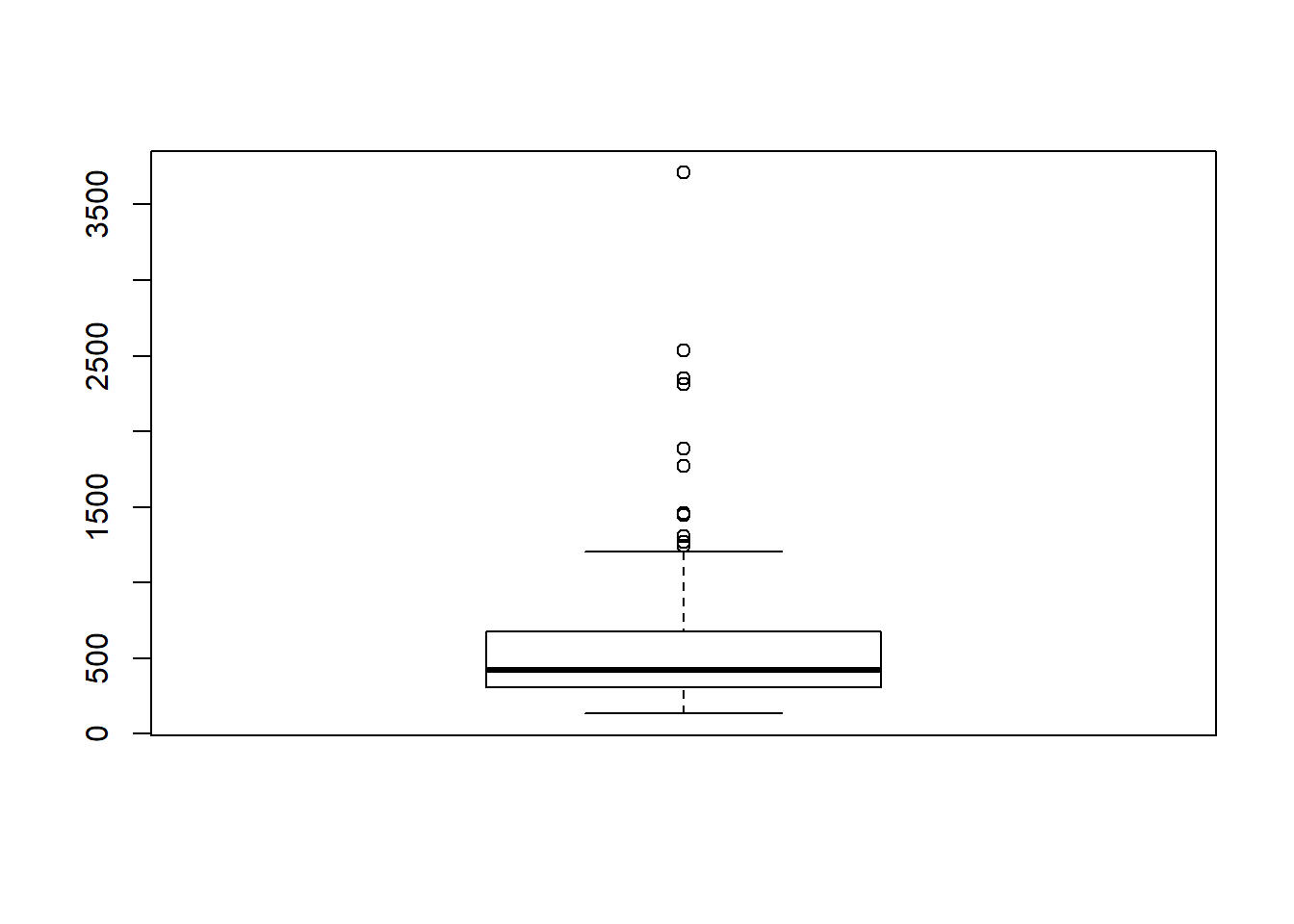# b.
# Distribution seems strongly skewed to the right.

# c.
rivers_class <- cut(rivers, breaks = c(min(rivers), 250, 500, 750, 1000, 1250, max(rivers)), include.lowest = TRUE)

# d.
barplot(table(rivers_class))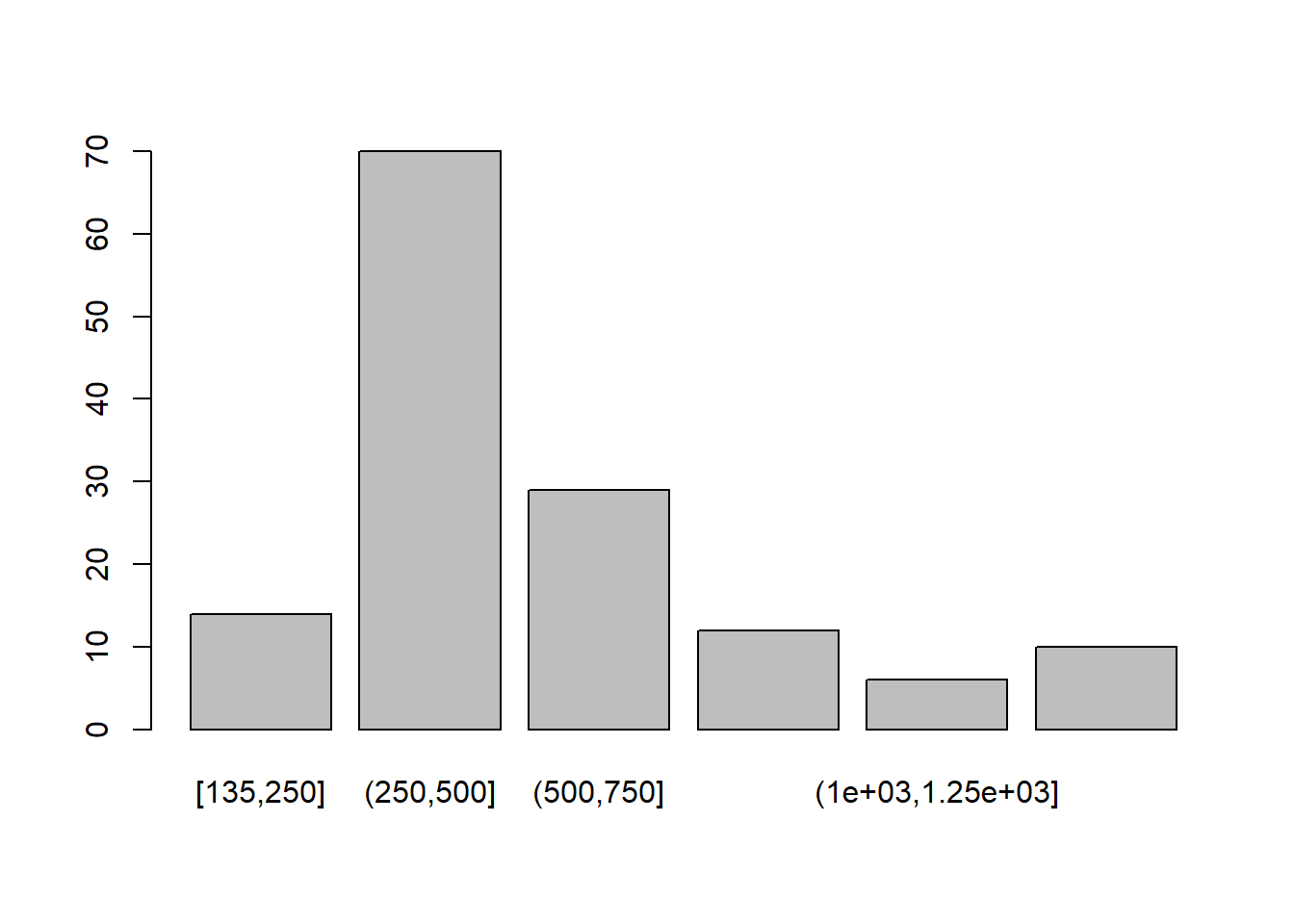# e.
# Depends on the purpose but the barplot at least shows the main bulk of the data more clearly.

1. The data set islands contains the areas of all landmasses in the world which exceed a certain threshold.
1. Find a suitable way to visualize the data and plot it.
2. How are the landmasses distributed based on your plot?
3. Compute both robust and non-robust measures of location and scatter for the data.
4. Remove some of the outliers (and think of a possible reason for justifying this!) from the data and compute the same measures as in part c.
5. Compare the results of part c and part d.
# a.
# Not very informative due to the outliers.
# Maybe some better options exist...
hist(islands)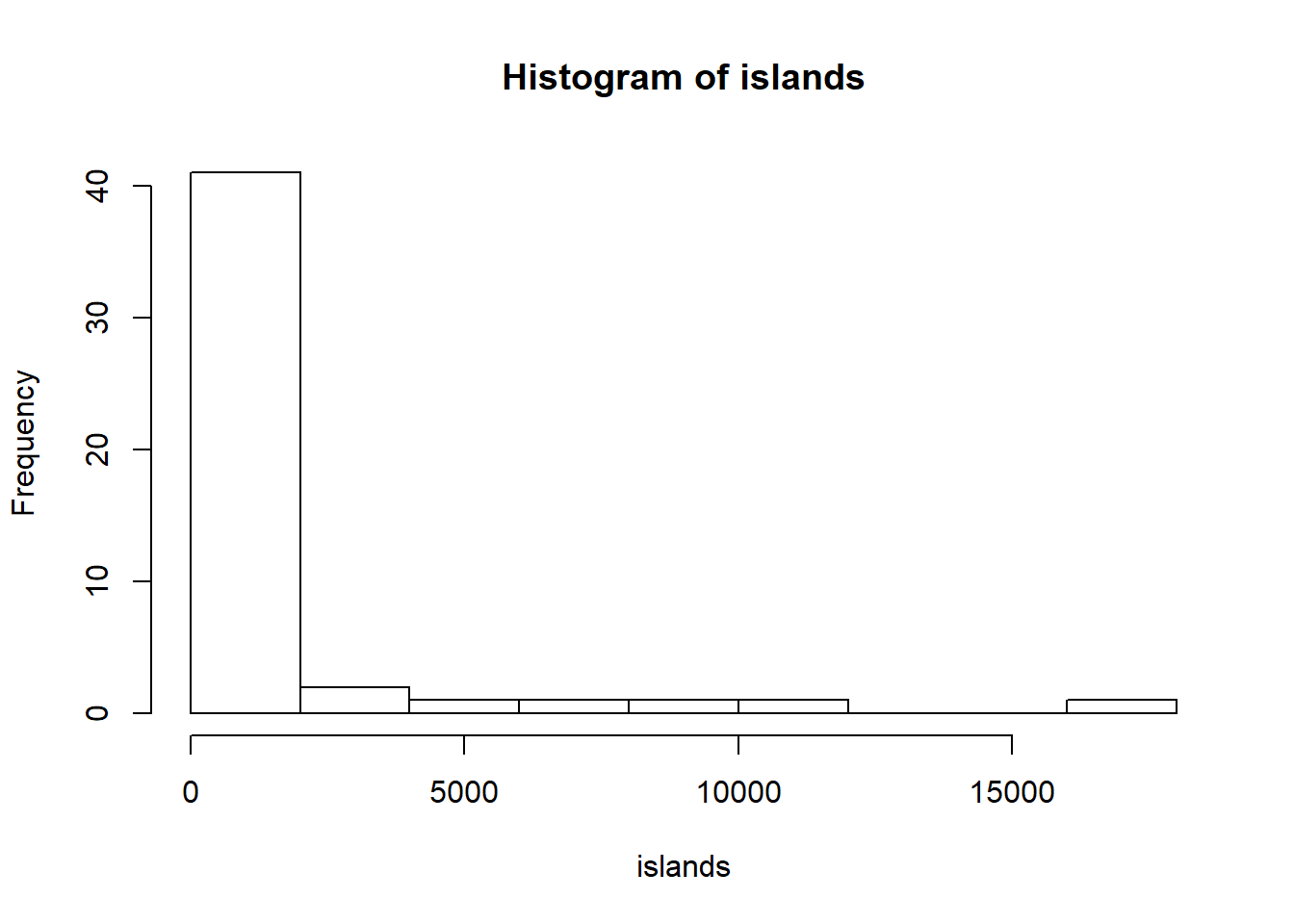# Log-transform de-emphasizes the outliers somewhat.
hist(log(islands))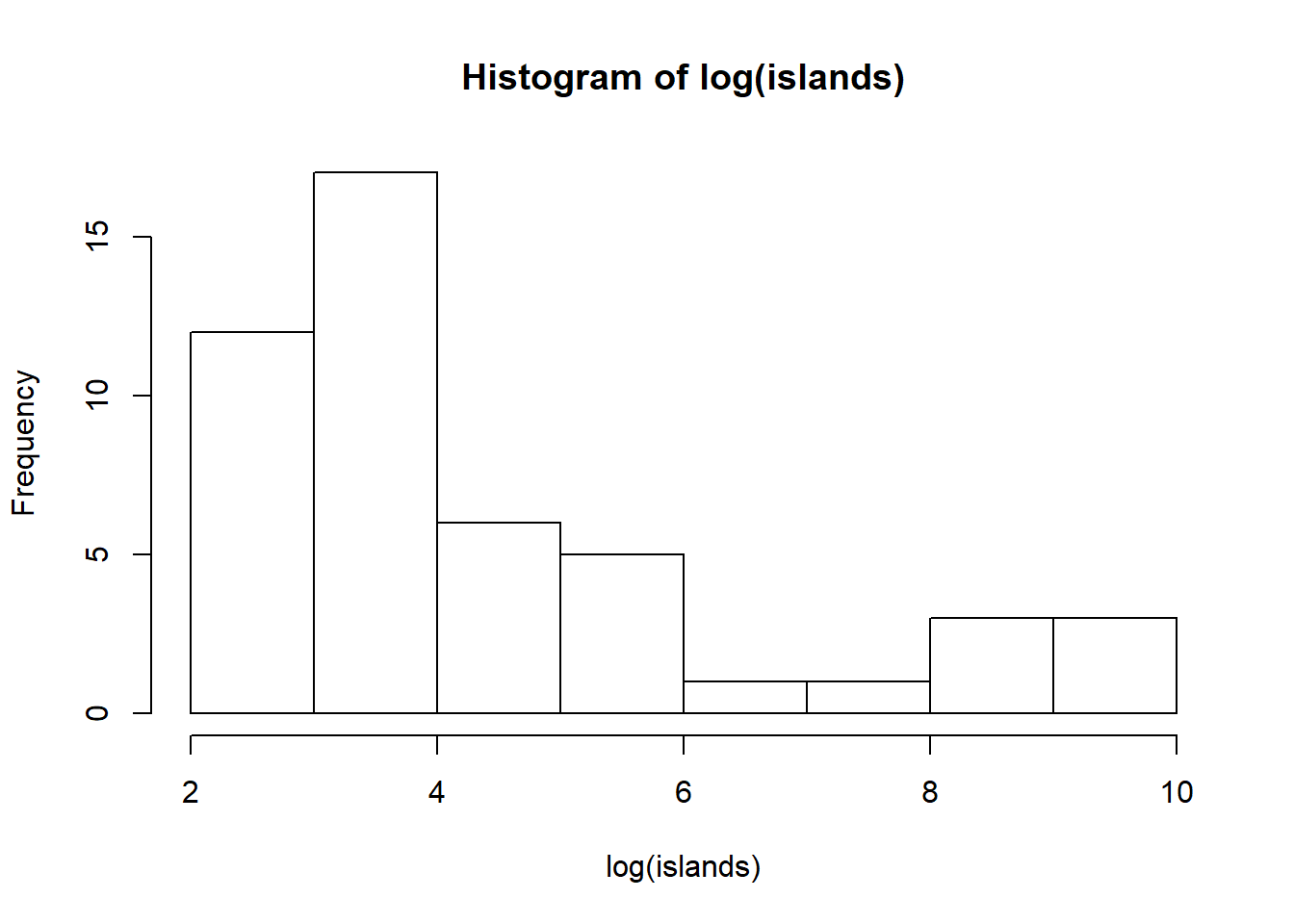# b.
# The distirbution is strongly skewed to the right.

# c.
# Location
mean(islands)
##  1252.729
median(islands)
##  41
# Scatter
sd(islands)
##  3371.146
mad(islands)
##  39.2889
# d.
# Maybe we are only interested in the non-continent landmasses and remove all the continents
islands_2 <- islands[-order(islands, decreasing = TRUE)[1:7]]

# Location
mean(islands_2)
##  79
median(islands_2)
##  32
# Scatter
sd(islands_2)
##  141.5035
mad(islands_2)
##  25.2042
# e.
# The non-robust measures of location and scatter changed proportionally a lot more when removing the "outliers" than the robust measures.

1. The data set Nile contains yearly measurements of the flow of the river Nile.
1. Find a suitable way to visualize the data and plot it.
2. How has the flow of the river changed during the years 1871-1970 based on the plot?
3. Calculate the values of the following statistics for the flow: mean, standard deviation, variance, minimum, maximum, median, median absolute deviation, mode, skewness and kurtosis.
4. How are each of the statistics in part c visible in the plot of part a?
# a.
# The data are time series and thus time should be a part of the plot
# "Nile" is already saved as a time series object in R and the plain "plot" command produces a plot of flow vs. time.
plot(Nile)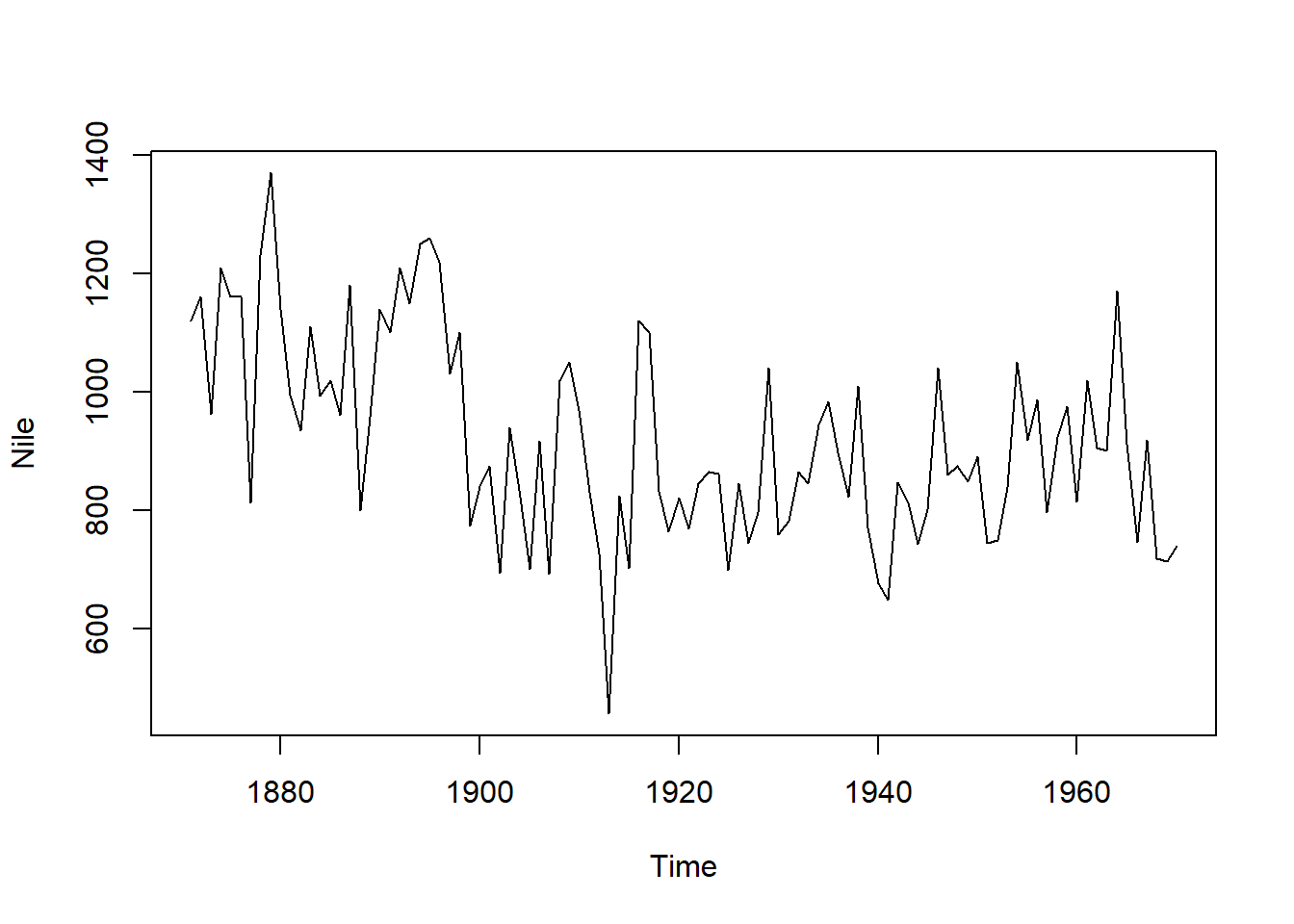# b.
# The data seems to fluctuate (seasonally?). During the first 40 years of measurement there was a general downward trend after which the flow has stayed around a fixed level.

# c.
mean(Nile)
##  919.35
sd(Nile)
##  169.2275
var(Nile)
##  28637.95
min(Nile)
##  456
max(Nile)
##  1370
median(Nile)
##  893.5
mad(Nile)
##  179.3946
# All four first values below are modes
sort(table(Nile), decreasing = TRUE)
## Nile
##  845 1020 1100 1160  744  874 1040 1050 1120 1140 1210  456  649  676  692
##    3    3    3    3    2    2    2    2    2    2    2    1    1    1    1
##  694  698  701  702  714  718  726  740  742  746  749  759  764  768  771
##    1    1    1    1    1    1    1    1    1    1    1    1    1    1    1
##  774  781  796  797  799  801  812  813  815  821  822  824  831  832  833
##    1    1    1    1    1    1    1    1    1    1    1    1    1    1    1
##  838  840  846  848  860  862  864  865  890  897  901  906  912  916  918
##    1    1    1    1    1    1    1    1    1    1    1    1    1    1    1
##  919  923  935  940  944  958  960  963  969  975  984  986  994  995 1010
##    1    1    1    1    1    1    1    1    1    1    1    1    1    1    1
## 1030 1110 1150 1170 1180 1220 1230 1250 1260 1370
##    1    1    1    1    1    1    1    1    1    1
library(moments)
skewness(Nile)
##  0.3223697
kurtosis(Nile)
##  2.695093
# d.
# Mean = the average level around which the data fluctuates
# SD & Var = the average size of these fluctuations
# Min = the lowest point of the curve
# Max = the lowest point of the curve
# Median = the average level around which the data fluctuates (robust)
# MAD = the average size of these fluctuations (robust)
# (Mode = difficult to see...)
# (Skewness = difficult to see...)
# (Kurtosis = difficult to see...)

1. (Optional) Try out the 3d-visualization tools in the package rgl. The following code plots an interactive 3d-scatter plot of the first three variables in the iris data. Find out how you can colour the points in the plot according to the variable Species.
install.packages("rgl")
library(rgl)

# Opens in a new window
open3d()
plot3d(iris[, 1:3])

1. (Optional) Pretty plots are often cumbersome to produce with base R and numerous packages offer various more attractive approaches. Try out the package ggplot2 by running the following code.
install.packages("ggplot2")
library(ggplot2)

ggplot(data = mpg, aes(x = hwy, y = cty)) +
geom_point() +
labs(x = "Highway miles per gallon", y = "City miles per gallon") 

What does the plot represent? Experiment with the code and find out how you can color the points according to the class of the car.

Numerous tutorials about ggplot2 can be found online. Check out at least https://r4ds.had.co.nz/data-visualisation.html and http://r-statistics.co/ggplot2-Tutorial-With-R.html.